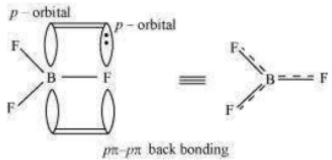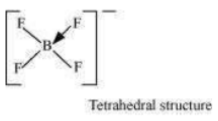Suggest reasons why the B–F bond lengths in $B{F}_{3}$ (130 pm) and $B{{F}_{4}}^{-}$(143 pm) differ.

The B–F bond length in $B{F}_{3}$ is shorter than the B–F bond length in$B{{F}_{4}}^{-}$  . $B{F}_{3}$ is an electron-deficient species. With a vacant p-orbital on boron, the fluorine and boron atoms undergo pπ–pπ back-bonding to remove this deficiency. This imparts a double bond character to the B–F bond.This double-bond character causes the bond length to shorten in $B{F}_{3}$ (130 pm). However, when $B{F}_{3}$ coordinates with the fluoride ion, a change in hybridisation from $s{p}^{2}$ (in $B{F}_{3}$) to

$s{p}^{3}$
(in $B{{F}_{4}}^{-}$) occurs. Boron now forms 4σ bonds and the double-bond character is lost. This
accounts for a B–F bond length of 143 pm in $B{{F}_{4}}^{-}$ ion.